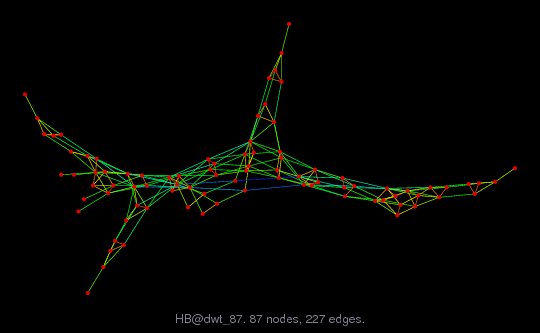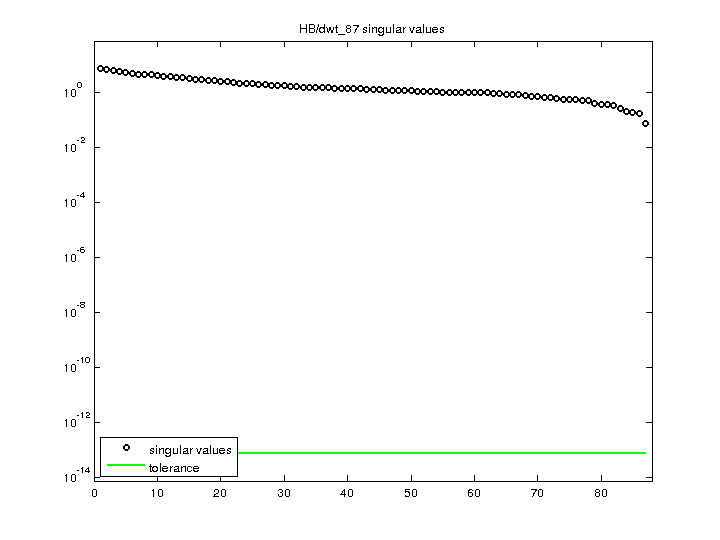Matrix: HB/dwt_87

Description: SYMMETRIC CONNECTION TABLE FROM DTNSRDC, WASHINGTON(undirected graph drawing)• Matrix group: HB
• download as a MATLAB mat-file, file size: 1 KB. Use UFget(136) or UFget('HB/dwt_87') in MATLAB.

 Matrix properties number of rows 87 number of columns 87 nonzeros 541 structural full rank? yes structural rank 87 # of blocks from dmperm 1 # strongly connected comp. 1 explicit zero entries 0 nonzero pattern symmetry symmetric numeric value symmetry symmetric type binary structure symmetric Cholesky candidate? yes positive definite? no

 author G. Everstine, D. Taylor editor I. Duff, R. Grimes, J. Lewis date 1980 kind structural problem 2D/3D problem? yes

 Ordering statistics: result nnz(chol(P*(A+A'+s*I)*P')) with AMD 414 Cholesky flop count 2.2e+03 nnz(L+U), no partial pivoting, with AMD 741 nnz(V) for QR, upper bound nnz(L) for LU, with COLAMD 525 nnz(R) for QR, upper bound nnz(U) for LU, with COLAMD 921

 SVD-based statistics: norm(A) 7.71355 min(svd(A)) 0.076215 cond(A) 101.208 rank(A) 87 sprank(A)-rank(A) 0 null space dimension 0 full numerical rank? yes

 singular values (MAT file): click here SVD method used: s = svd (full (A)) ; status: ok